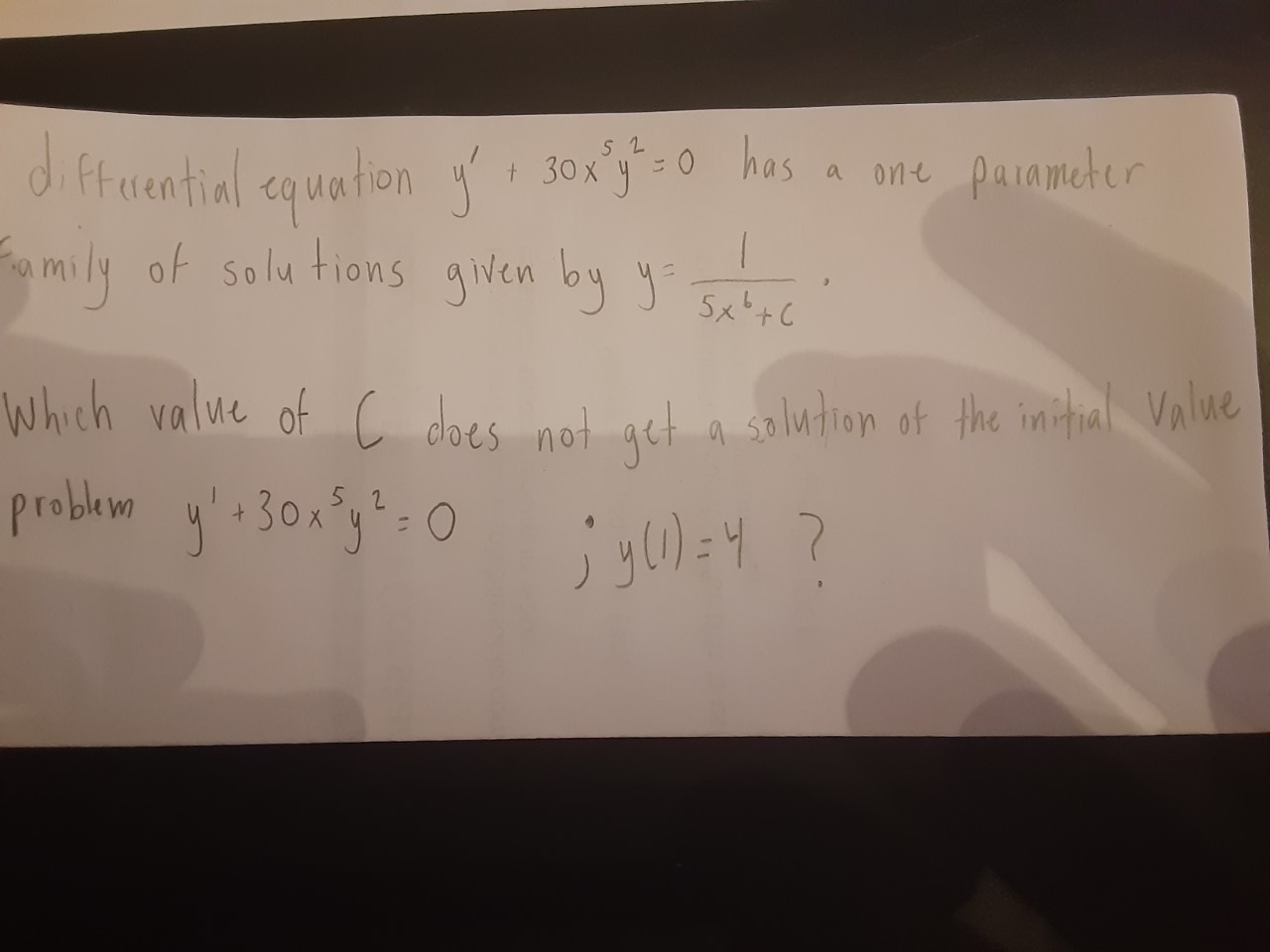# 3ox'y 0 hasdifruential equationd ftaential equationmily of solu tions given byhos a ane painmeterJox'so+ 30x 45x+CWhich value of cproblem y:30xg=0L dloes not get a solution of the imitial Value%3Djyu)=4 ?

Question
14 viewshelp_outlineImage Transcriptionclose3ox'y 0 has difruential equation d ftaential equation mily of solu tions given by hos a ane painmeter Jox'so + 30x 4 5x+C Which value of c problem y:30xg=0 L dloes not get a solution of the imitial Value %3D jyu)=4 ? fullscreen
check_circle

Step 1

For the given differential equation, the...

### Want to see the full answer?

See Solution

#### Want to see this answer and more?

Solutions are written by subject experts who are available 24/7. Questions are typically answered within 1 hour.*

See Solution
*Response times may vary by subject and question.
Tagged in

### Math